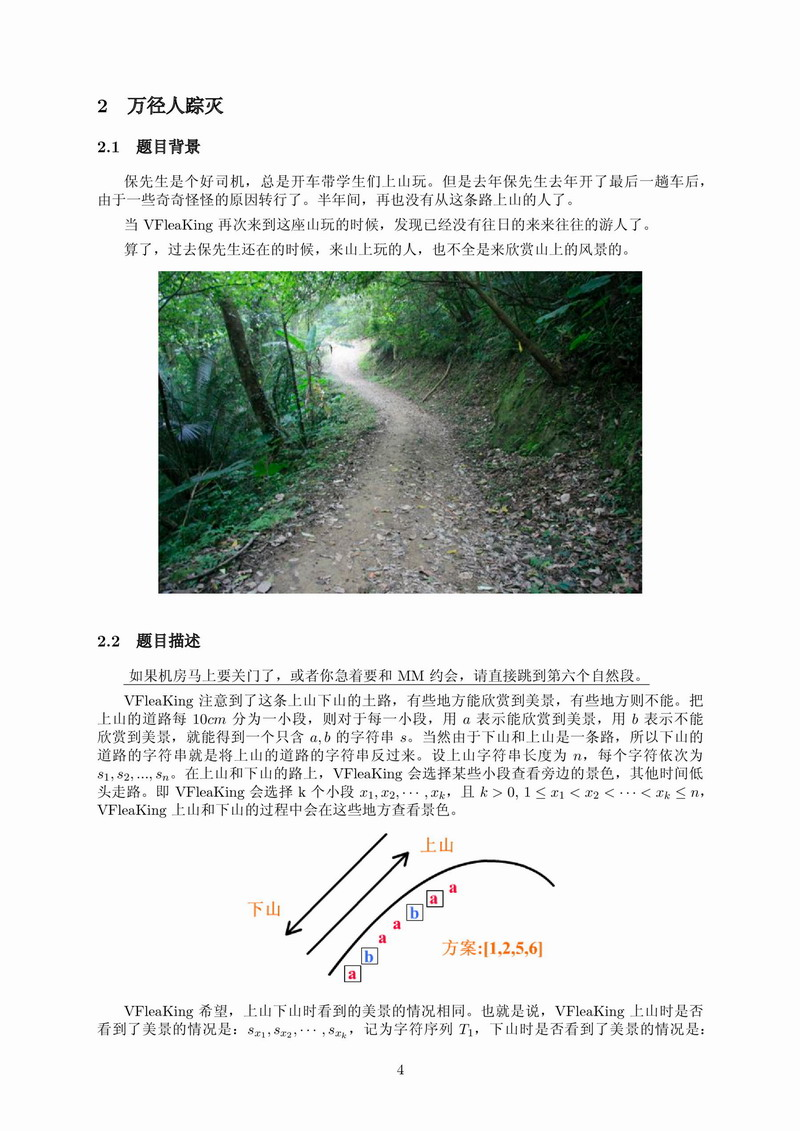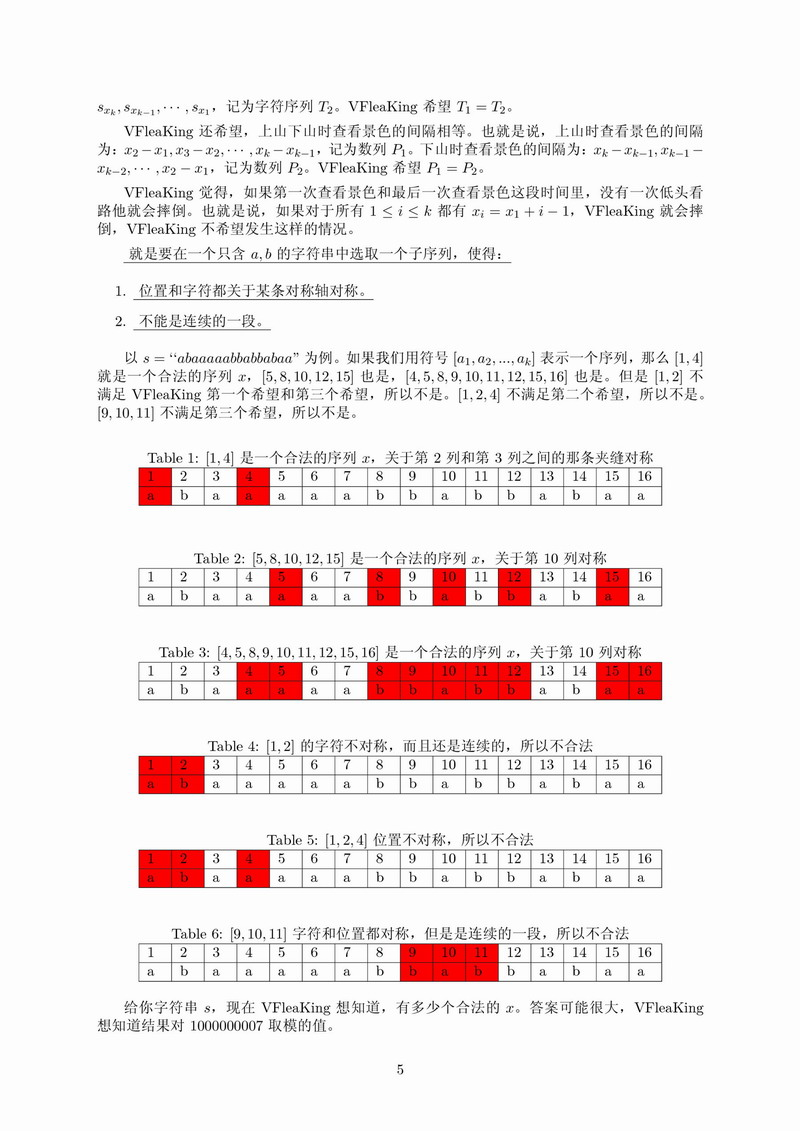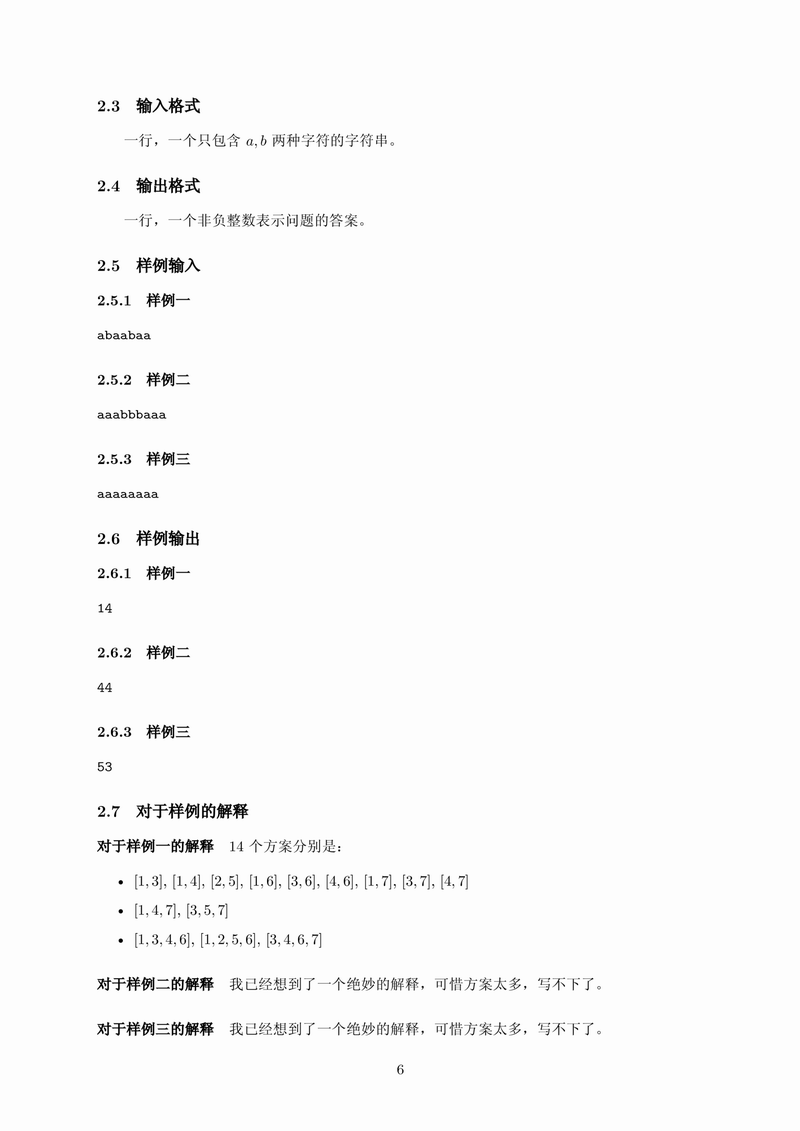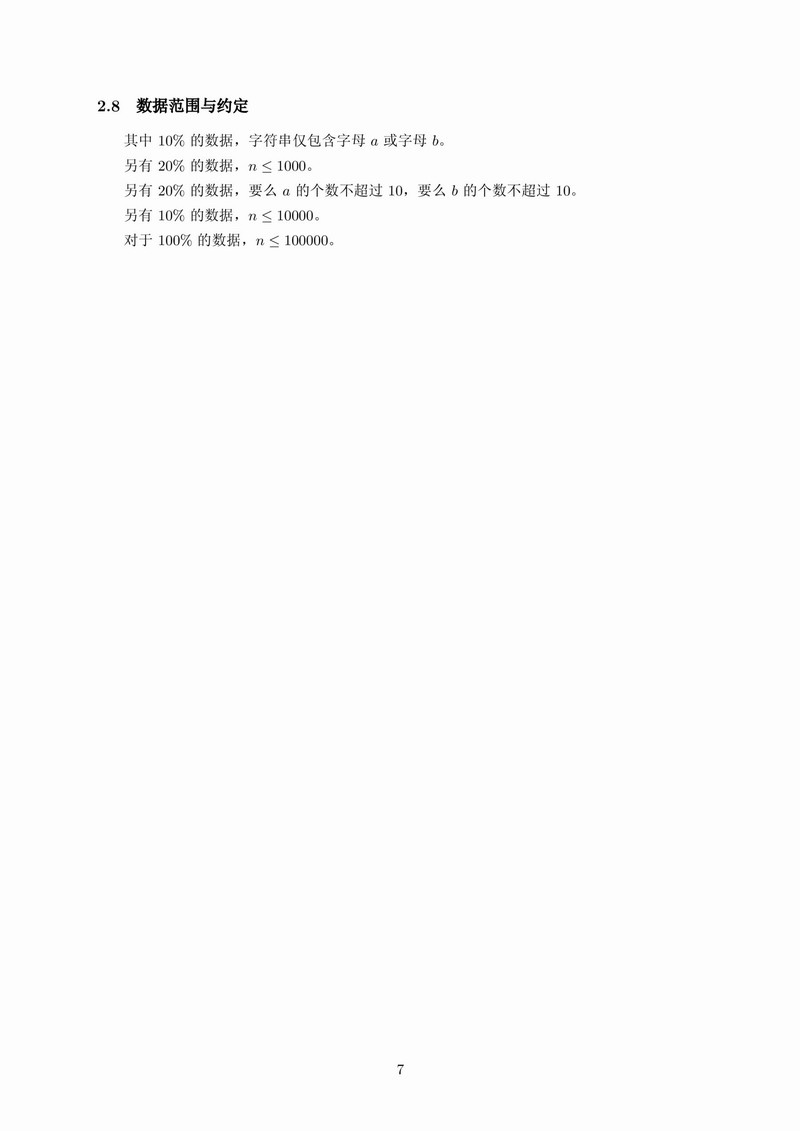DescriptionInput&OutputSolution

从对称轴入手还是挺好想的啊>_<。

题目要求所有不连续对称回文子序列。那我们就求出所有对称回文子序列，然后减去连续的情况。连续的情况可以跑一次Manacher求出。

现在问题转换成如何求回文子序列。

我们考虑一条对称轴$x=0.5k,\, k \in N \cap [0,2(n-1)]$，以$x$为对称轴能产生许多回文子序列。

不难发现，回文子序列满足下列性质：对于每个回文子序列中的字符$s_i​$，必定存在$j​$，使得$s_i=s_j,\, i+j=2x​$。而且$s_j​$也一定在回文子序列上。因此每个回文子序列是由多个$(i,j)​$二元组组成的，且二元组满足$s_i=s_j, \, i + j = 2x​$。

换言之，如果定义$f(2x)$表示以$x$为对称轴时，满足$s_i=s_j, \, i+j=2x$的二元组$(i,j)$个数，那么这条对称轴对答案的贡献为$2^{f(2x)}-1$。

现在想想怎么求$f(x)$。

根据$f(x)$的定义，可得$f(x)=\sum_{i+j=x}[s_i==s_j]=\sum_{i=0}^{x}[s_i==s_{x-i}]$

看着像卷积？然而不会解。。。

我们可以换个思路，由于字符串中只有$a$和$b$，因此可以分别考虑$a$和$b$对对称轴$x$的贡献。

由于$a$和$b$的问题是一样的，不妨只考虑$a$。

对对称轴产生贡献的前提是$s_i==s_j$和$i+j=2x$，由于限定了$a$，因此条件一满足。现在就是求解有多少个二元组$(i,j)$满足$i+j=2x$。

就是说，有很多个下标，要求选择2个，使得和为$2x$。这个不就是生成函数的常见例题吗。。。

因此构造出生成函数，然后FFT加速就可以解决啦。